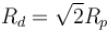Courses

# Magnetostatics MCQ Level - 1

## 10 Questions MCQ Test Topic wise Tests for IIT JAM Physics | Magnetostatics MCQ Level - 1

Description
This mock test of Magnetostatics MCQ Level - 1 for Physics helps you for every Physics entrance exam. This contains 10 Multiple Choice Questions for Physics Magnetostatics MCQ Level - 1 (mcq) to study with solutions a complete question bank. The solved questions answers in this Magnetostatics MCQ Level - 1 quiz give you a good mix of easy questions and tough questions. Physics students definitely take this Magnetostatics MCQ Level - 1 exercise for a better result in the exam. You can find other Magnetostatics MCQ Level - 1 extra questions, long questions & short questions for Physics on EduRev as well by searching above.
QUESTION: 1

Solution:

QUESTION: 2

### A magnetic field of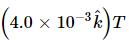exerts a force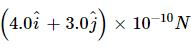on a particle having a charge 10−9C and moving in te x−y plane. Find the velocity of the particle.

Solution:

Given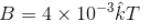q = 10–9 C
Magnetic force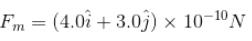Let velocity of the particle in x-y plane be,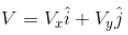Then from the relation
Fm =  q(V × B)
We have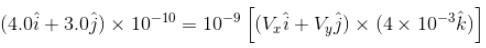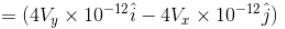Comparing the coefficient of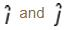we have,
4 × 10–10 = 4Vy × 10–12
Vy = 102 m/s = 100 m/s
and  3.0 × 10–10 = –4Vx × 10–12
Vx = –75 m/s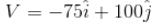The correct answer is: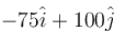QUESTION: 3

### The dimensional formula of magnetic induction is :

Solution: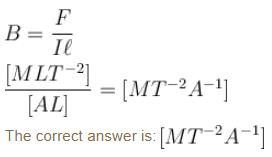QUESTION: 4

If a charge particle is describing a circle of radius r  in a magnetic field with a time period T. Then :

Solution: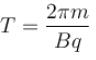So, T  is independent of  r.
The correct answer is: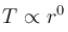QUESTION: 5

Two wires of same length are shaped into a square and a circle. If they carry same current, ratio of the magnetic moment is

Solution:

Let length of each wire is  L
For square length of each side  L/4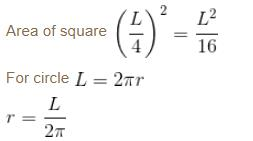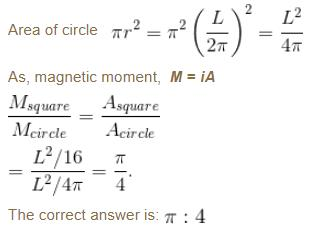QUESTION: 6

A positively charged particle falling freely under gravity enter a region having a uniform horizontal magnetic field pointing north to south the particle will be deflected towards

Solution:

Given that , V is pointing downwards and B is towards North

As magnetic force in given by,

f​m=q(V×B)

Using right hand thumb for negative charge we get the F is towards west

QUESTION: 7

A charged particle enters a magnetic field H with its initial velocity making an angle of 45° with H the path of the particle will be :

Solution:

The correct answer is: a helix

QUESTION: 8

A wire carrying current i and other carrying current 2i  in the same direction produce a magnetic field B at the mid-point. What will be the field when 2i current is switched off?

Solution:

Magnetic field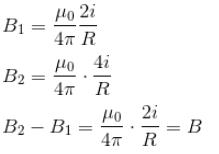B2 = 2B1
2B1 – B1 = B
B1 = B

QUESTION: 9

A long metallic cylinder of radius R has a current i flowing through it. The magnetic induction B  will vary with distance from the curve as shown in figure.

Solution: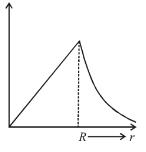QUESTION: 10

A proton and a deutron both having the same kinetic energy, enter perpendicularly into a uniform  magnetic field B. For motion of proton and deutron on circular path of radius Rp and Rd  respectively, the correct statement is :

Solution:

For charged particle on circular path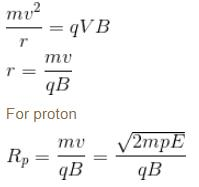For deutron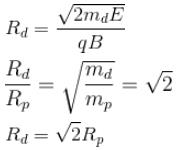The correct answer is: